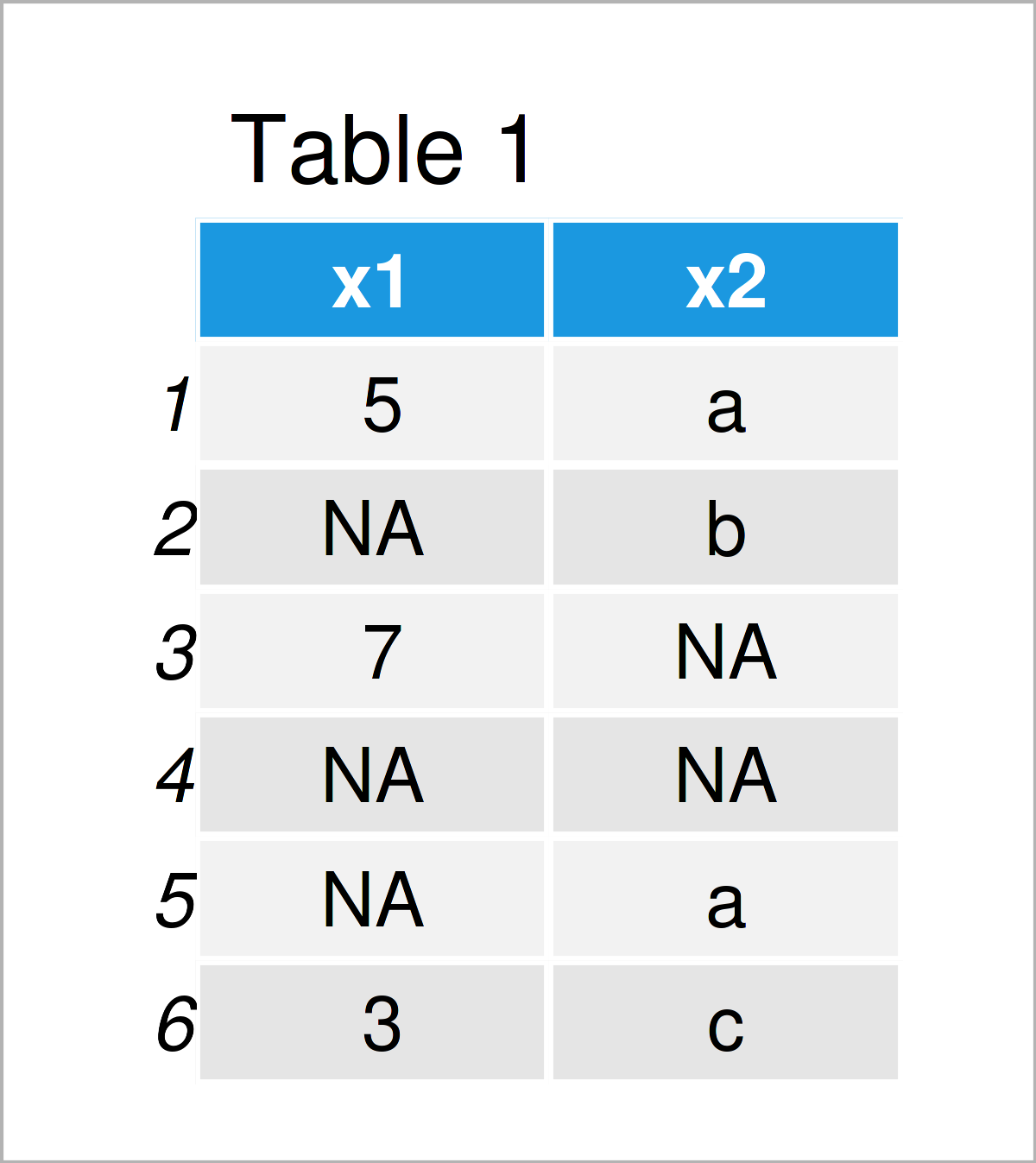# Count Non-NA Values in R (2 Examples)

In this article you’ll learn how to get the number of non-NA values in R programming.

The tutorial will contain this information:

Let’s get started.

## Example 1: Count Non-NA Values in Vector Object

In Example 1, I’ll demonstrate how to find the number of non-missing values in a vector object.

For this example, we first have to create an exemplifying vector:

```vec <- c(1, NA, 2, NA, NA, 1, 2, 1, NA)            # Create example vector
vec                                                # Print example vector
#   1 NA  2 NA NA  1  2  1 NA```

Next, we can apply the sum and is.na functions to this vector to get the number of non-NA values:

```sum(!is.na(vec))                                   # Get number of non-NA values
#  5```

As you can see based on the previous output of the RStudio console, our vector object contains five non-NA values.

## Example 2: Count Non-NA Values in Columns & Rows of Data Frame

In this example, I’ll explain how to return the number of non-NA values in a data frame.

Let’s create some example data:

```data <- data.frame(x1 = c(5, NA, 7, NA, NA, 3),    # Create example data frame
x2 = c("a", "b", NA, NA, "a", "c"))
data                                               # Print example data frame```By executing the previous code, we have managed to construct Table 1, i.e. a data frame containing six rows and two columns. Both of the columns contain some NA values.

If we want to count the non-NA values in each column of this data frame, we can apply the colSums and is.na functions as shown below:

```colSums(!is.na(data))                              # Get number of non-NA values in columns
# x1 x2
#  3  4```

The column x1 contains three non-NA values, and the column x2 contains four non-NA values.

It is also possible to count the non-NA values by rows. For this, we have to exchange the colSums function by the rowSums function:

```rowSums(!is.na(data))                              # Get number of non-NA values in rows
#  2 1 1 0 1 2```

The previously shown vector shows the number of non-NA values in each of the six rows in our data frame.

## Video & Further Resources

Do you need more info on the topics of this article? Then I recommend having a look at the following video on my YouTube channel. In the video, I explain the contents of this article in the R programming language:

Please accept YouTube cookies to play this video. By accepting you will be accessing content from YouTube, a service provided by an external third party.If you accept this notice, your choice will be saved and the page will refresh.

In addition, you might want to read some of the related articles on my homepage.

In this article you have learned how to count the number of non-NA values in the R programming language. If you have any additional questions, kindly let me know in the comments section.

Subscribe to the Statistics Globe Newsletter